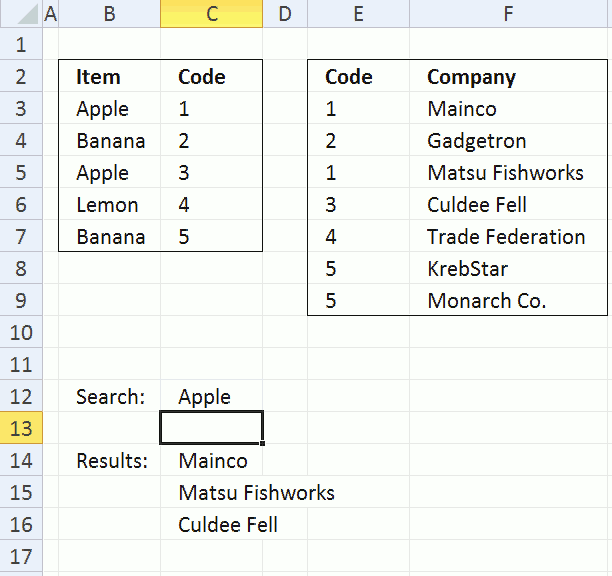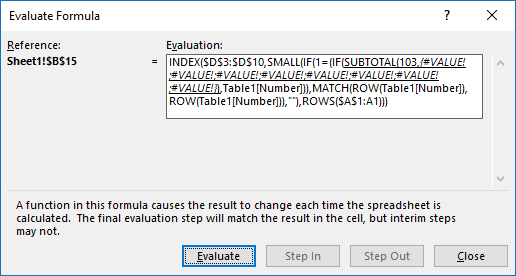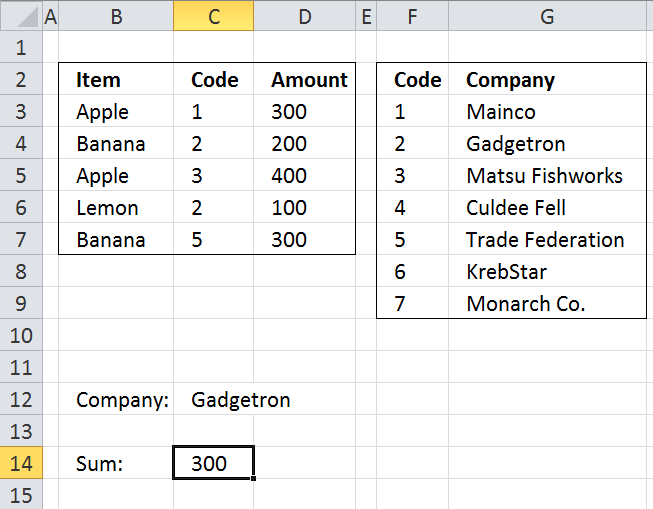Author: Oscar Cronquist Article last updated on October 17, 2019Excel 2010 has a PowerPivot feature and DAX formulas that let you work with multiple tables of data. You can connect tables to each other based on relationships. When relationships are made nothing stops you from doing lookups to related values and relational tables or sum values for a relational table.

#### What is a relational table?

In a relational database (Microsoft Access), the data in one table is related to the data in other tables. In general, tables can be related in one of three different ways: one-to-one, one-to-many or many-to-many.  The relationship is used to cross-reference information between tables.
Source: University of Sussex

This post is not about PowerPivot and DAX formulas, it is about doing lookups in two tables and they have one column in common. This means that the columns contain the same values, however, not necessarily in the same order. This makes the two data sets related because they share a value.

The image above shows a formula in cell C14 that looks for a value, specified in cell C12, in B3:B7. The corresponding value in C3:C7 is then used do a lookup in E3:E9. The formula then returns the corresponding values in F3:F9 to C14:C16. This is possible because they share the same values in column C and in column E.

Here is an example, cell C12 contains Apple, Apple is found in cell C3 and C5. The corresponding values in C3:C7 are 1 and 3. The formula looks for 1 and 3 in E3:E9 and finds cell E3, E5 and E6. Now the formula returns values from the same rows to C14:C16, the values are "Mainco", "Matsu Fishworks" and "Culdee Fell".

I'll also demonstrate a formula that sums values in a relational table.

### Search for values in a related tableThe animated image above explains how the concept works. The following formula can return a single value but it returns multiple values if more values match. Not only does it match multiple values in the first table, it also matches multiple values in the related table as well.

Array formula in cell C14:

=IFERROR(INDEX(\$F\$3:\$F\$9, SMALL(IF(ISERROR(MATCH(\$E\$3:\$E\$9, IF(\$C\$12=\$B\$3:\$B\$7, \$C\$3:\$C\$7, ""), 0)), "", MATCH(ROW(\$F\$3:\$F\$9), ROW(\$F\$3:\$F\$9))), ROW(A1))), "")

How to create an array formula

1. Select cell C14
2. Copy above array formula
3. Press with left mouse button on in formula bar
4. Paste array formula
5. Press and hold Ctrl + Shift
6. Press Enter

How to copy array formula

1. Select cell C14
2. Copy (Ctrl + c)
3. Select cell range C15:C17
4. Paste (Ctrl + v)

### Explaining array formula in cell C14

#### How can I examine formula calculations in greater detail?

You can follow formula calculations quite easily using the "Evaluate Formula" feature in Excel. Select cell C14 and then go to tab "Formulas" on the ribbon. Press with mouse on "Evaluate Formula" button to open an "Evaluate" dialog box.(The formula shown above in the Evaluate Formula" dialog box is not used in this article.)

Press with left mouse button on "Evaluate" button to see the next calculation step. I have demonstrated these calculations steps below.

#### Step 1 - Search for a value

The IF function has three arguments. IF(logical_test, [value_if_true], [value_if_false])

The logical_test argument contains an expression that either returns TRUE or FALSE, in this case the value in cell C12 is compared to all values in cell range B3:B7.

The corresponding value in cell range C3:C7 is returned if the expression returns TRUE and nothing is returned if FALSE.

IF(\$C\$12=\$B\$3:\$B\$7, \$C\$3:\$C\$7, "")

becomes

IF("Apple"={"Apple"; "Banana"; "Apple"; "Lemon"; "Banana"}, {1; 2; 3; 4; 5}, "")

and returns {1;"";3;"";""}.

#### Step 2 - Use column in common to find matches

The MATCH function returns a number representing the relative position if a value exists in a cell range or array. It returns #N/A if not found.

MATCH(\$E\$3:\$E\$9, IF(\$C\$12=\$B\$3:\$B\$7, \$C\$3:\$C\$7, ""), 0)

becomes

MATCH(\$E\$3:\$E\$9, {1;"";3;"";""}, 0)

becomes

MATCH({1; 2; 1; 3; 4; 5; 5}, {1;"";3;"";""}, 0)

and returns

{1;#N/A;1;3;#N/A;#N/A;#N/A}

#### Step 3 - Return row numbers

The ISERROR function is used to identify error values in the array, the IF function replaces error values with a blank "" and numbers with the corresponding row number.

IF(ISERROR(MATCH(\$E\$3:\$E\$9, IF(\$C\$12=\$B\$3:\$B\$7, \$C\$3:\$C\$7, ""), 0)), "", MATCH(ROW(\$F\$3:\$F\$9), ROW(\$F\$3:\$F\$9)))

becomes

IF(ISERROR({1;#N/A;1;3;#N/A;#N/A;#N/A}, "", MATCH(ROW(\$F\$3:\$F\$9), ROW(\$F\$3:\$F\$9)))

becomes

IF(ISERROR({1;#N/A;1;3;#N/A;#N/A;#N/A}, "", {1; 2; 3; 4; 5; 6; 7})

and returns {1; ""; 3; 4; ""; ""; ""}

#### Step 4 - Return a value of the cell at the intersection of  a particular row and column

The corresponding row number is used by the INDEX function to return a specific value based on row and column numbers. The SMALL function extracts a row number based on a relative cell reference and the ROW function. The relative cell reference changes when the cell is copied and pasted to cells below.

=INDEX(\$F\$3:\$F\$9, SMALL(IF(ISERROR(MATCH(\$E\$3:\$E\$9, IF(\$C\$12=\$B\$3:\$B\$7, \$C\$3:\$C\$7, ""), 0)), "", MATCH(ROW(\$F\$3:\$F\$9), ROW(\$F\$3:\$F\$9))), ROW(A1)))

becomes

=INDEX(\$F\$3:\$F\$9, SMALL({1; ""; 3; 4; ""; ""; ""}, ROW(A1)))

becomes

=INDEX(\$F\$3:\$F\$9, 1)

becomes

=INDEX({"Mainco"; "Gadgetron"; "Matsu Fishworks"; "Culdee Fell"; "Trade Federation"; "KrebStar"; "Monarch Co."}, 1)

and returns Mainco in cell C14.

### Sum values in a related tableArray formula in cell C14:

=SUM(IF(ISERROR(MATCH(\$C\$3:\$C\$7, IF(\$C\$12=\$G\$3:\$G\$9, \$F\$3:\$F\$9, ""), 0)), 0, \$D\$3:\$D\$7))

How to create an array formula

### Get the Excel fileSearch-related-tables-array-formula.xlsx